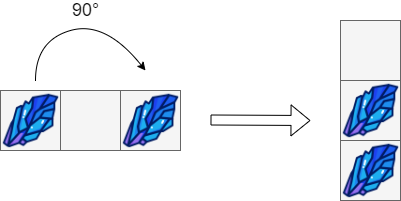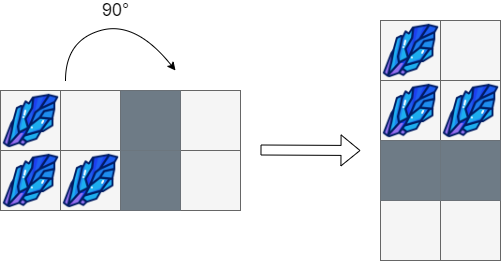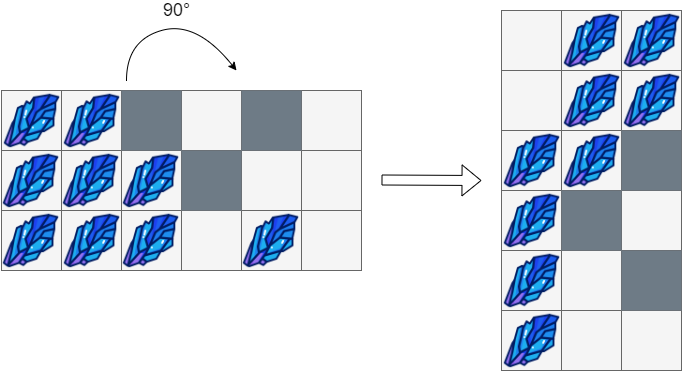Formatted question description: https://leetcode.ca/all/1861.html

# 1861. Rotating the Box

Medium

## Description

You are given an m x n matrix of characters box representing a side-view of a box. Each cell of the box is one of the following:

• A stone '#'
• A stationary obstacle '*'
• Empty '.'

The box is rotated 90 degrees clockwise, causing some of the stones to fall due to gravity. Each stone falls down until it lands on an obstacle, another stone, or the bottom of the box. Gravity does not affect the obstacles’ positions, and the inertia from the box’s rotation does not affect the stones’ horizontal positions.

It is guaranteed that each stone in box rests on an obstacle, another stone, or the bottom of the box.

Return an n x m matrix representing the box after the rotation described above.

Example 1:Input: box = [["#",".","#"]]
Output: [["."],
["#"],
["#"]]


Example 2:Input: box = [["#",".","*","."],
["#","#","*","."]]
Output: [["#","."],
["#","#"],
["*","*"],
[".","."]]


Example 3:Input: box = [["#","#","*",".","*","."],
["#","#","#","*",".","."],
["#","#","#",".","#","."]]
Output: [[".","#","#"],
[".","#","#"],
["#","#","*"],
["#","*","."],
["#",".","*"],
["#",".","."]]


Constraints:

• m == box.length
• n == box[i].length
• 1 <= m, n <= 500
• box[i][j] is either '#', '*', or '.'.

## Solution

First, move the stones to the rightmost positions considering the border of the box and the obstacles. Then rotate box 90 degrees clockwise.

class Solution {
public char[][] rotateTheBox(char[][] box) {
int m = box.length, n = box.length;
moveToRight(box);
char[][] rotated = new char[n][m];
for (int i = 0; i < m; i++) {
for (int j = 0; j < n; j++)
rotated[j][m - 1 - i] = box[i][j];
}
return rotated;
}

public void moveToRight(char[][] box) {
int m = box.length, n = box.length;
for (int i = 0; i < m; i++) {
int prevObstacle = n;
int stones = 0;
for (int j = n - 1; j >= 0; j--) {
if (box[i][j] == '*') {
int k = prevObstacle - 1;
while (k > j && stones > 0) {
box[i][k] = '#';
k--;
stones--;
}
while (k > j) {
box[i][k] = '.';
k--;
}
prevObstacle = j;
} else if (box[i][j] == '#')
stones++;
}
if (stones > 0) {
int k = prevObstacle - 1;
while (k >= 0 && stones > 0) {
box[i][k] = '#';
k--;
stones--;
}
while (k >= 0) {
box[i][k] = '.';
k--;
}
}
}
}
}